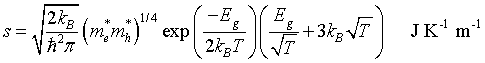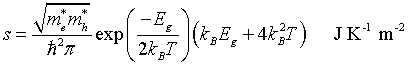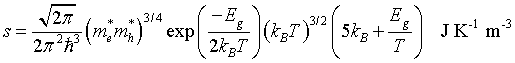## Boltzmann approximation

The table below gives the contribution of electrons in intrinsic semiconductors and insulators to some thermodynamic quantities. These results where calculated in the Boltzmann approximation where it is assumed that the chemical potential lies in the band gap more than 3kBT from the band edge. The electronic contribution to the thermodynamic quantities are usually much smaller than the contribution of the phonons and thus the electronic components are often simply ignored.

 1-d 2-d 3-d Density of states me* and mh* are 'density of states' effective masses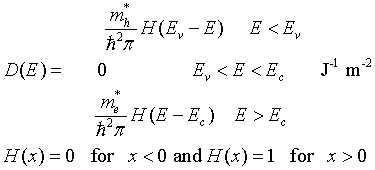Density of states Nv and Nc are the effective densities of states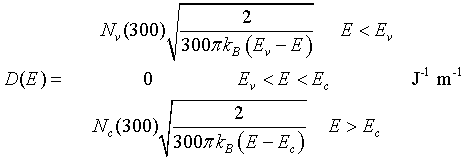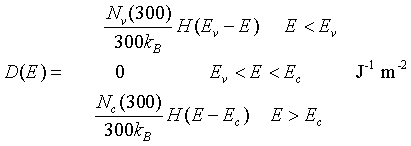Density of electrons in the conduction band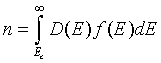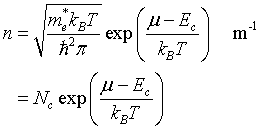Density of holes in the valence band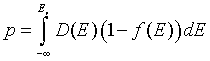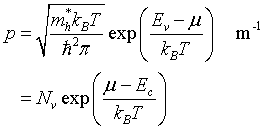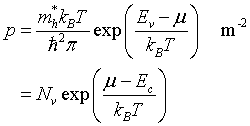Law of mass action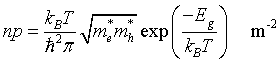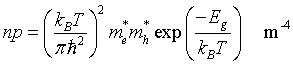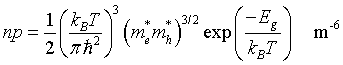Intrisic carrier density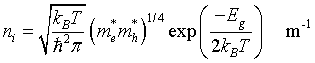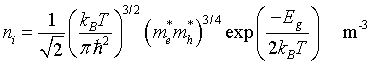Chemical potentialSet n = p, solve for μ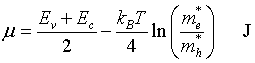Internal energy density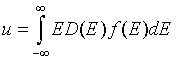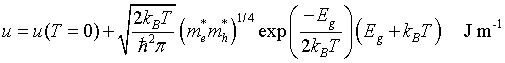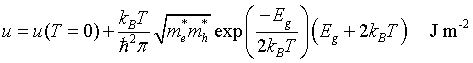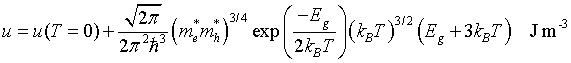Helmholtz free energyf = u - Ts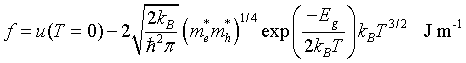Specific heat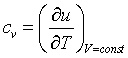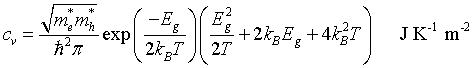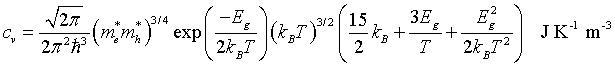Entropy